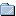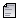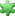###Author Topic: Vortrex's Helpful Snippets  (Read 2133 times)

#### Vortrex« on: March 14, 2013, 05:27:57 pm »
Here's a couple of snippets for everybody to enjoy.

First, here's a function to return the vector in front of a vector. All you need to calculate this is the current position to get the front of, the angle to calculate, and the distance away from the current position. This function can be used on both server and client side (depending on what script you put it in).
Code: [Select]
`function GetVectorInFrontOfVector(vector,angle,distance){ angle = angle * PI / 180; local x = (vector.x + ((cos(angle + (PI/2)))*distance)); local y = (vector.y + ((sin(angle + (PI/2)))*distance)); return Vector(x,y,vector.z);}`
Below is the same type of function, but is used to get a vector behind a vector.
Code: [Select]
`function GetVectorBehindVector(vector,angle,distance){ angle = angle * PI / 180; local x = (vector.x + ((cos(-angle + (PI/2)))*distance)); local y = (vector.y + ((sin(-angle + (PI/2)))*distance)); return Vector(x,y,vector.z);}`

• Tester
• Full Member
•• Posts: 144
• Karma: +16/-35« Reply #1 on: March 15, 2013, 04:59:32 pm »
Why do people always have to create new topics....

http://forum.liberty-unleashed.co.uk/index.php/topic,1594.msg9154.html#msg9154

If you have useful snippets, post them over here.#### Vortrex